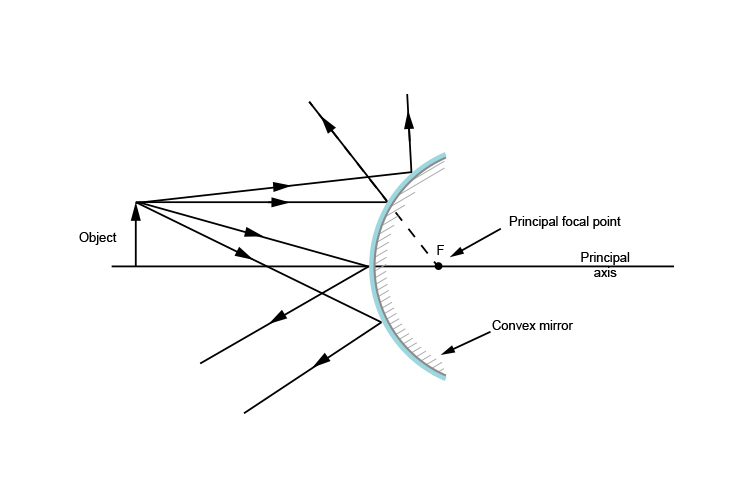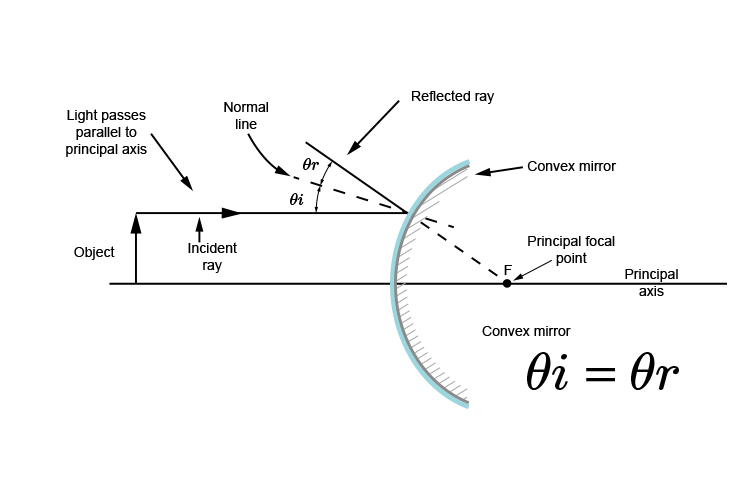# Which is the principal focal point – Convex mirror

Many rays of light reflect off every point of an object, but which is the ray of light which tells you where the principal focal point is?Again we emphasise that the principal focal point is where a ray which is parallel to the principal axis appears to cross the principal axis behind the mirror.This parallel light ray that bounces off the convex mirror can be projected behind the convex mirror. Where it crosses the principal axis is the principal focal point.